﻿ Foundation of Complex Matter Space and Special Theory of Relativity, a Unifying ApproachJournal of Nuclear and Particle Physics

p-ISSN: 2167-6895    e-ISSN: 2167-6909

2014;  4(5): 147-153

doi:10.5923/j.jnpp.20140405.03

### Foundation of Complex Matter Space and Special Theory of Relativity, a Unifying Approach

Reza Ahangar

Department of Mathematic, Texas A & M University-Kingsville, Kingsville, USA

Correspondence to: Reza Ahangar, Department of Mathematic, Texas A & M University-Kingsville, Kingsville, USA.
 Email: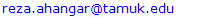Abstract

Relativistic mechanics cannot recognize speed faster than light. In this article, a new fundamental view on matter will be presented and the paradigm will be shifted from real or pure imaginary particle to Complex Matter Space. We will investigate the relativistic concept of Complex Matter Space. A new assumption will be imposed that matter has two intrinsic components, i) mass, and ii) charge. The mass will be measured by a real number and charge by an imaginary unit. We are hoping that this approach will help us to present a relativistic view of energy and momentum in Complex Matter Space. We will conclude that the new Complex Matter Space (CMS) theory will help get one step closer to a better understanding toward, 1) the conversion of mass and energy 2) Energy and Momentum, 3) unifying the forces, 4) tachyon, dark energy & dark matter, and also antiparticles.

Keywords: Complex Matter, complex Energy, and Complex Momentum

Cite this paper: Reza Ahangar, Foundation of Complex Matter Space and Special Theory of Relativity, a Unifying Approach, Journal of Nuclear and Particle Physics, Vol. 4 No. 5, 2014, pp. 147-153. doi: 10.5923/j.jnpp.20140405.03.

### 1. Complex Matter a New View of Imaginary Mass and Charges

Using Doppler Shift phenomena, scientists can learn much about the motion of galaxies. It is known that the light from one side of the galaxy is blue shifted and the light from the other side is red shifted. This indicates that one side is moving toward the earth, and the other is moving away. All these will help us to determine the speed at which the galaxy is rotating. Using the frequency of the rotation can help estimate masses in galaxies. Dark energy was first proposed in 1998 when two groups of astronomers made a survey of exploding stars or supernovas in distant galaxies. These cosmological observations strongly suggest that the universe is dominated by dark energy. Both dark energy and dark matter are not distinct, but are a unique entity of matter (Shibili -2007).
Recent observations by NASA agree that dark energy and dark matter represent one entity and have densities close to the range 10-26 kg/m3. The ratio between energy density (in Joules) and the mass density (kg/m3) are proportional to the square of the speed of light. (Shibli 2006 & 2007).
The content of the paper on "Imaginary Relativity" is a right direction toward Complex Matter Space (CMS) but not sufficient for the unifying forces (Rezaei 2010). The CMS postulates may answer some of the questions in this article's concluding remarks. This could be a reason for the statement "the author does not know where the imaginary world is located or what its nature is?".
It is important to postulate that matter has two components that can be demonstrated in a complex plane as a real component and an imaginary component: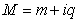(1.1)
Both papers (Mayer 2013) and (Zhang 2008) considered their models in complex planes but did not include matter of particles as a complex variable. Many authors attempted to unify the electromagnetic force and gravitational force.
With this assumption, we may have more challenges to verify the interactions of electromagnetic and gravitational forces by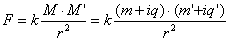(1.2)
We can observe that, after expansion of (1.2), two terms on the right hand side represent Gravitational forces and Electromagnetic forces. But two other imaginary components seemed to me not to represent other forces that need to be justified.
Many physicists including Neils Bohr (Wheeler 1983), Richard Feynman (Feynman 1963) have presented their computational models using complex numbers.
This extension to complex number systems occurred in the time-dependent Schrodinger equation and in Dirac and Von Neumann's state vector approach. They occur in both the state vectors themselves and often also with the operators on them.
According to the original postulate, we determine a point in a complex plane by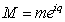. One can explore and study the implication of this assumption, and demonstrate the consistency with other phenomenon, theories, or experiments.
The existence of a particle traveling faster than light has been presented by many authors including, Sudarshan 1970 who discussed the Physics of complex mass.
The organization of this paper is the following: A new View of complex matter will be presented based on two new postulates. A geometric interpretation of Complex Matter will be used to justify them in a complex coordinate plane.
By these new assumptions of Mass and Energy, Newtonian Mechanics will be restructured in section two. In section three we will observe Newtonian Mechanics in Complex Matter Space and challenges in Imaginary Mass, Force, Acceleration, and Momentum.
In this relativistic view of Complex Matter, Einstein's equation of mass-energy will be revised.
The relativistic view of the complex Matter Space will be investigated in section four. In section five the derivation of Einstein's equation in complex matter space will be presented. The relativistic view of energy will be discussed in section six.
Finally, energy and momentum will be recalculated in the Complex space. In section seven, we will explore a possible explanation of the Existence of Negative Matter and Antimatter, as well as dark energy and dark matter. Further possible investigation and research will be presented.

### 2. Overview of Complex Matter SPACE (CMS)

Assume that the terminology "matter" in general is an undefined term. In this paper, in addition to the postulates of Special Relativity Theory (SRT) we accept the following new postulates:
Postulate (I): mass and charge are two intrinsic components of matter and they can be measured, described, and determined in a complex plane. The real part is equal to the mass and the imaginary part is the charge of the particle. That is M=m+iq.
Postulate (II):
In one dimensional space, position and velocity are assumed to be real numbers.
By accepting the second postulate of CMS we are admitting that the particle can be selected in a complex plane and the velocity of a particle can be assumed to be faster than the speed of light.
The position x(t) and the velocity dx/dt will stay a real number. The speed of light remains a constant real number, c. By this agreement, and contrary to the paper "Imaginary Relativity" by Rezaei (Rezaei 2010), we are not accepting variation of the speed of light or considering it as a complex number.
In addition the complex plane of (t,E) is not an ordered set. Thus, using the Heisenberg Principle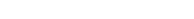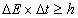should be adjusted to a real number.
For decades, the discovery of the nature of other forces was a dream.
Acceptance of this postulate leads to the necessity to reaffirm the entire physics, Newtonian Mechanics, Relativity, and Quantum mechanics.

#### 2.1. Pure Imaginary Unit for Time in 4D–Space

Absolute time may be an undefined word and we try to postulate, define, and measure it by its changes, variations, and motions with other physical quantities in space. Physical motion of particles, rotation of the earth around the sun, or clock's hands on a circle. The unit of time practically is what we perceive about how much physical objects change position, velocity, energy, or mass. How do we define one hour, one minute, or one second? They are the measurements of the physical changes that we try to demonstrate by arc-length on a clock using the unit of distance or radians on a circle.
Are they real measurements of the time? In the following mathematical argument we introduce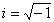as a unit of time on the imaginary axis.
Let us consider a vector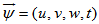such that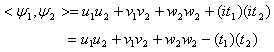(2.1)
Magnitude of a Vector 4D-Complex Matter Space: We may use the norm in the inner product space as a distance or magnitude of a vector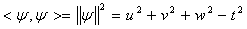#### 2.2. Verifying the CMS Postulate (II)

According to time and length dilation in SRT, the value of time and length variation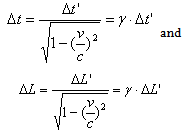(2.2)
such that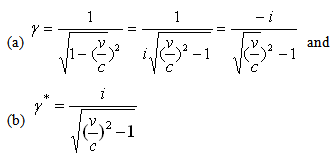(2.3)
can be interpreted for the velocity v as a real number greater than the speed of light (see Taylor 2004).
This is one indication that time may have an imaginary unit.
Assuming a complex time variation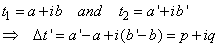where we replaced, p=a’-a, q=b’-b. The relation (2.2) can be expressed byCase (I): the motion velocity v<c.
The values of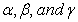will remain real numbers. Consequently according to (2.2) velocity v and distance L will be real numbers.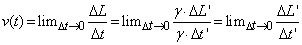Case (II): If the particle velocity is faster than the speed of light (v>c) then both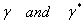are pure imaginary numbers and using (2.8) the following show that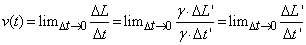is a real number.
This argument infers that the inequality v>c demonstrates the relation in an ordered set. This is not true in the complex plane (see Naber 1992).

### 3. Complex Matter in Newtonian Mechanics

Having a new unified presentation of mass and charge in the form of Complex Matter will bring a new question and challenges in force, acceleration, energy, and momentum. How do we interpret the principles of Newtonian Mechanics?
a) Newton's First: If no net force acts on a body its acceleration is zero.
It is assumed that when an object is under the influence of a force, if the object is at rest it stays at rest and if it is in motion the motion will be uniform.
Further investigation is required to clarify the terminology of undefined term and consistency with other Newtonian mechanics.
b) Newton's Second Law: It is proven that many different Forces exerted on an object cause changes in the position of the object and create a variety of accelerations in the motion and of the object.
The second of Newton's Laws of motion of an object will be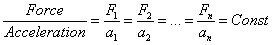(3.1)
This constant real number in Newtonian classical mechanics is named "m=mass". This terminology in Complex Matter may need to be changed to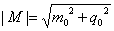and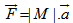where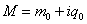is in the complex plane, m0 is mass at rest, and q0 is the charge of the initial particle.
The alternative way to express Newton's Law for complex matter will be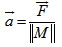(3.2)
This law for gravitational fields will be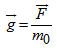and for electric fields will be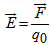.
The unit dimension of g is usually written as meters/sec2, but could be written as nt/kg. The unit dimension for E is written as (Newtons/ Coulomb) nt/coul.
Example: A particle of mass m and charge q is placed at rest in a uniform electric field m and released. Its motion can be described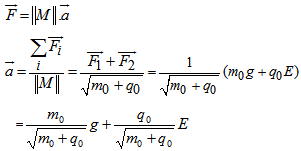The unit for the measurement is a linear combination of g and E. This process is the resultant of two vectors of gravitation and charge.
Traveling Beyond the Speed of Light: We may not be allowed to cross the boundary of the speed of light c in the real world. However, if we can pretend that we are existing in the imaginary world then we are allowed to go faster than light, since our conjugate space will be the real world.
Assume that we are traveling by a rocket which is taking off from the earth and wishes to go cruising in space. How much energy is required to accelerate to the speed of light? it would be impossible for a particle to be accelerated to c because the speed of light is constant in a vacuum for all inertial observers. What do we observe? i) Observers in all inertial frames on the earth would measure the same value for c and in fact infinite energies are required to accelerate objects to the speed of light.
ii) If we can break the wall of speed of light and travel faster than light, an observer would see things going crazy around – by SRT time dilation the time would freeze around us, - mass will approach infinity.

### 4. Relativistic View of the Complex Matter SPACE

Antimatter: A substance composed of elementary particles having the mass and electric charge of ordinary matter (such as electrons and protons) but for which the charge and related magnetic properties are opposite in sign. The existence of antimatter was posted by the electron theory of P.A.M. Dirac. In 1932 the positron (antielectron) was detected in cosmic rays, followed by the antiproton and the antineutron detected through the use of particle accelerators. Positrons, antiprotons, and antineutrons, collectively called antiparticles, are the antiparticles of electrons, protons, and neutrons, respectively. When matter and antimatter are in close proximity, annihilation occurs within a fraction of a second, releasing large amounts of energy. The (relativistic) mass of an object measured by an observer in the xyz-frame is given by(4.1)
By accepting the complex matter Postulate (I), the velocity in (4.1) can be any real or complex number. Mathematically v could be greater than the speed of light, but the mass M0 would become imaginary. Physically we would have to get to the speed of light first i.e. v=c, which gives us an undefined value for M. However, if we believe that nothing moves faster than the speed of light because we do not like observables objects to be imaginary?
I) A hypothetical particle that is traveling equal or faster-than-light (photon, or tachyon) is consistent with the special theory of relativity. According to this theory, a free particle has an energy E and a momentum p which form a Lorentz four-vector. The length of this vector is a scalar, having the same value in all inertial reference frames. One writes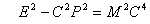(4.2)
Eq. (4.2), where c is the speed of light and the parameter M2 is a property of the particle, independent of its momentum and energy. Three cases may be considered: M2 may be positive, zero, or negative. The case M2 > 0 applies for atoms, nuclei, and the macroscopic objects of everyday experience. If M2 = 0, the particle is called massless. A few of these are known: the electron neutrino, the muon neutrino, the photon, and the graviton. The name tachyons (after a Greek word for swift) has been given to particles with M2 < 0.
Mayer 2007, designed the energy momentum in a complex plane very well without acknowledging the idea of complex matter considering the speed of light c=1 a natural unit. The relation (4.2) can be interpreted by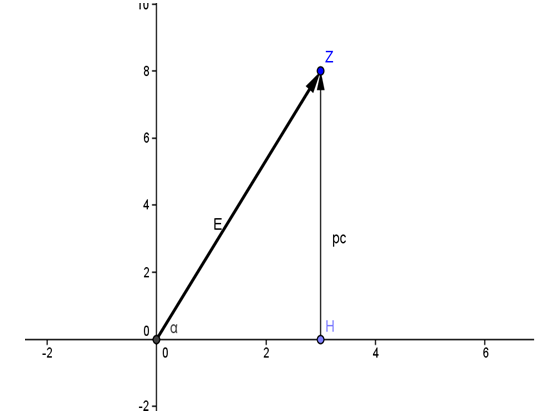Figure (1). Energy-momentum in the Complex plane. A vector form of the energy demonstrated with the horizontal component OH=mc2, and vertical component ZH=pc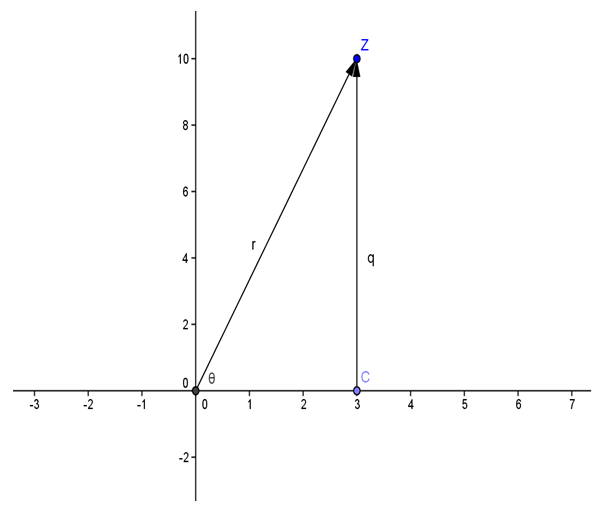Figure (2). The state of the Complex Matter in a complex plane with two components (m,q), where m is the real part of the "matter" and q is the imaginary part
The point M(m,q) in Fig.(2) is in the complex plane and it is denoted by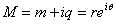, where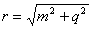, and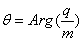.
In Fig (1), a vector form of the energy demonstrated with the horizontal component OH=mc2, and vertical component ZH=pc. Energy vector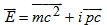with a magnitude can be expressed by: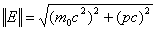.
The portraits in Fig.(1) and Fig.(2) demonstrate the implication of postulate (I) to the state of the Energy. That is, energy can be expressed with two components of real and imaginary values. The Energy of a mass-less particle can be observed on the diagram when,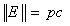. When the real part of particle mass is not zero we will derive the energy, mass, and charge in relativistic mechanics.
The complex point E can be described in the following form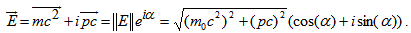(4.3)

### 5. Derivation of Einstein's Equation in Complex Matter Space

Using Postulate (I) let us describe a particle of a complex Matter Space by a set of points M(t)=m(t)+iq(t) where m is the mass and q is the charge at time t in the interval (a,b) for local parameters a and b.
We will describe the instantaneous rate of changes of this complex matter by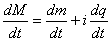.
Lemma (5.1): Given a complex matter in (4.1) then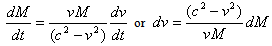(5.1)
Proof: Assuming M=m+iq and taking the derivative of (4.1)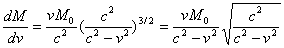This concludes the relation (5.1).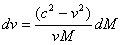Theorem (5.1): The instantaneous rate of change in complex matter can be described by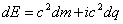(5.2)
Proof: Let us assume in the following approach, that the definition of energy and force for the complex matter space is staying the same. Thus;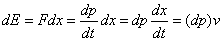(5.3)
where, the velocity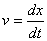is a real vector function but the momentum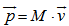will be a complex vector function. The differentiation of momentum will be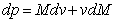(5.4)
Substitute in (5.3)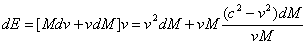Simplifying the right hand side concludes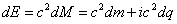(5.5)
The Energy can be computed when time t is in the interval [a,b]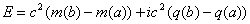To explain the relativistic energy in Complex Matter Space, we use the trigonometric values in the complex plane;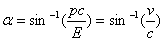and assume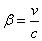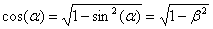When the real part of particle mass is not zero we will derive the energy, mass, and charge in relativistic mechanics.
The complex point E can be described in the following form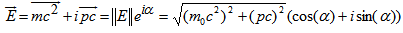Replacing cosine and sine of angle alpha from the previous step, we will get an energy state description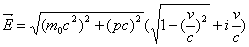(5.6)
This is a correction of the conclusion presented in a paper by (Myer 2007). The relation (5.6) is the state of the energy of a particle with the real rest mass moving faster than light.
Momentum of Complex Matter: Assume that M0=(m0,q0) represents two components of a particle at rest. Moving at the velocity v(t)=dx/dt on the real axis, x will be a real function of time. The momentum at time t will be### 6. Relativistic View of Energy in Complex Matter Space

I) Energy Expression: Complex Matter space will be able to interpret the duality of nature mass-wave characteristic matter. On the other hand, there are some quantum mechanical solutions that allow negative masses like the expression for relativistic energy-momentum.
Let's try to re-investigate the quantum mechanical view of the SRT in Complex Matter Inner Space. This will allow us to consider mass M=m+iq. Thus, the relativistic model (1) will be in the following form:
Step (1): The famous Einstein relationship for Energy-Mass for a particle will be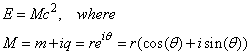(6.1)
Thus: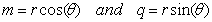(6.2)
The Complex Matter operations like M2 may need to be reinterpreted to fit the reality of physical nature. Thus the magnitude of the vector M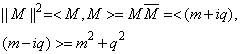could be a best way to explain the energy and momentum computations.
A free particle has a mass magnitude with Complex Matter in the Inner Space. By this property, 4.2 can be redefined by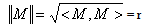(6.3)
Complex Matter having a magnitude in SRT, can be described by, at t=t0, for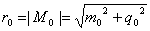and Complex Momentum can be described by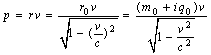(6.4)
for any velocity v. We will use the complex mass magnitude to evaluate the momentum p=||M||.vSubstitute: E=||M||c2 then,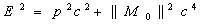(6.5)
II) Real and Imaginary Components of Energy: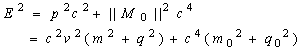(6.6)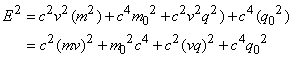Consequently the energy in the complex plane can be described by the magnitude of a vector with two components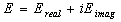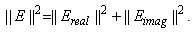(6.7)
As a conclusion, the magnitude of the energy is the sum of the magnitudes of real and imaginary energies.

### 7. Discussions

i)- Complex Matter Space, Dark Matter, and Dark Energy: If the value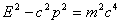is equal to zero then we have E=pc. Since c is a constant real number then m0 =0. This is the rest mass of a photon.
Indeed, that's also the reason why one can't really have a photon at rest, v=0. If the speed of a particle is c in one reference frame, it will stay c in any (non-singular) reference frame - that's another postulate of the special theory of relativity.
Consider M=m+iq such that the real part mass m will satisfy the following equation. When the rest mass of a photon is zero, that is m0 =0, then the relationfor v=c will be singular (0/0) but in a complex matter space in (4.1) for speed of a photon, v=c will not be singular.
The existence of a particle traveling faster than light has been presented by many authors including, Sudarshan 1970 who discussed the Physics of complex mass.
ii)-Complex Matter Space and Negative Mass: The idea of negative matter appears in past theories of matter that have now been abandoned. Using the once popular vortex theory of gravity, the possibility of matter with negative gravity was discussed by William Hicks in the 1880s.
In 1928, British physicist Paul A.M. Dirac generalized Einstein's famous equation E=mc² by considering negative mass. This hypothesis allowed for the existence of anti-particles in our universe
iii)- CMS explain Antiparticles: These anti-particles are, literally, mirror images of normal or real matter. Each anti-particle has the same mass as its corresponding particle, but the electrical charges are reversed. Examples of antimatter discoveries of the 20th century are: 1) Positrons - Electrons with a positive instead of negative charge, 2) Anti-protons - Protons that have a negative instead of the usual positive charge. 3) Anti-atoms - Pairing together positrons and antiprotons.
Both particles involved in the interaction process are completely annihilated and create a very huge explosion that occurs when antimatter and matter interact, transferring the entire mass of both objects into energy. It is part of the dream unification theory to build a matter-antimatter reaction engine?
iv)- Conclusions: The new Complex Matter postulate presented in this paper is the core foundation of this research. It was shown that with some modification it is consistent with Newtonian Mechanics. For speed less than the speed of light (v<c) similar modification demonstrates that the theory of complex matter is consistent with the Special Theory of Relativity. But for the speed faster than light then all relations and interpretations will be changed. In the complex matter space, the state of the energy and momentum and their magnitudes are presented in this paper.
v)- Future Research: To validate the conclusion of the complex matter space theory by experiment is beyond the scope of this paper. The author is humbly anxious to get help from those who are capable to perform these experiments for particles with velocity close but greater than the speed of light. The states of particles and their quantum states can be studied in Complex Matter Space through interactions with other fundamental particles.
The electromagnetic interaction, weak interaction forces, and strong Interaction forces can be considered. The standard model in quantum state does not consider Gravitation as part of the standard model.
The Complex Matter Space theory has a potential to apply to the interactions or collisions with particles generated in variety of forces.

### References

  Bibula 1996, Bkialnicki -Bibula, "Photon Wave Function," Progress in Optics 36, pp. 245-293 (1996).  Bohm 1993, David Bohm, The Undivided Universe (Routledge, London, 1993).  Dirac 1928, P.A.M. Dirac, "The Quantum Theory of Electron", Proc. R. Soc. A117, 610-624, p.619.  David Halliday and Robert Resnick with the Assistance of Farrell Edwards and John Merrill: "Fundamentals of Physics", John Wiley & Sons, Inc., 1970, SBN: 471-34430-3.  Feynman 1963, Richard Feynman, The Feynman Lectures on Physics (Addison Wesley, Reading, MA, 1963) Vol III, pp. 1-6, 7-5.  Gauthier 2009, Richard Gauthier, "The Dirac Equation and the Superluminal Electron Model",http://www.superluminalquantum.org, 2009.  Lord 1966, Lorch, Edgar Raymond, "Spectral Theory", Oxford University Press, Inc. Second Print 1966.  Mayer 2007, Alexander Franklin Mayer: "Wave Energy in Quantum Mechanics", Journal of Physics, Conference Series 70 (2007) 012013, IOP Publication:http://iopscience.iop.org/1712-6596/70/1/02013.  Naber 1992, Naber George L., "The Geometry of Minkowski Spacetime, An Introduction to the Mathematics of Special Theory of Relativity", Dover, 1992.  Rezaei 2010, Rezaei Mehran, "Imaginary Relativity", WSEAS TRANSACTION on COMMUNICATIONS, Issue 2, Volume 9, Feb. 2010, ISSN: 1109-2742, Esfahan Azad University, IRAN.  Shibili 2006, Murad Shibili, "The Equation of State of Dark Energy and Dark Matter: Boltzmann Constant and Unified Entity: The Utilization of Space Energy", College of Engineering, American University of Shrjeh, United Arab Emirates.  Shibili 2007, Murad Shibili: "The Foundations of the Fourth Law of Thermodynamics: Universe Dark Energy and Its Nature; Can Dark Energy be Generated?", International Conference on Renewable Energies and Power Quality, Seville Spain, March 26th-28th, 2007.http://www.icrepq.com/icrepq07/286-shibli.pdf  Sudarshan 1968, Sudarshan, E.C.G, "A New Formulation of Relativistic Quantum Theory of Fields with Applications to particles Traveling Faster than Light", Proc. Nobel Symposium on Elementary Particle Theory. Stockholm (1968). Also Proc. Indian Academy of Science 69A, 133 (1969).  Sudarshan, E.C.G, "Physics of Complex Mass Particles", Center for Particle Theory, Department of Physics, University of Texas, Austin TexasORO-3992-5, UT-26-0823-5, Jan. 1970.  Taylor 2004, John R. Taylor, Chris D. Zafirots, Michael A. Dubson, "Modern Physics for Scientists and Engineers", Physics Department University of Colorado Boulder, Second Edition, Pearson-Printice Hall, 2004.  Wall 1995, Wall Ernst. L., "The Vortex Theory of the Electron and its de Broglie Waves Pulse, ..", Institute for Basic Research, Palm Harbor, FL. www.gwphysics.com, 1995 and www.tachyonmodel.com.  Wheeler 1983, Wheeler John & Zurek Wojceich 1983: Neils Bohr, "the Quantum Postulate and the Recent Development of Atomic Theory," and John Wheeler and Wojceich Zurek, Quantum Theory and Measurement (Princeton: Princeton University Press, 1983), p. 111.  Zhang 2008, Zhang Tianxi, "Electric Charge as a form of Imaginary Energy", Progress in Physics, vol.2, 2008.